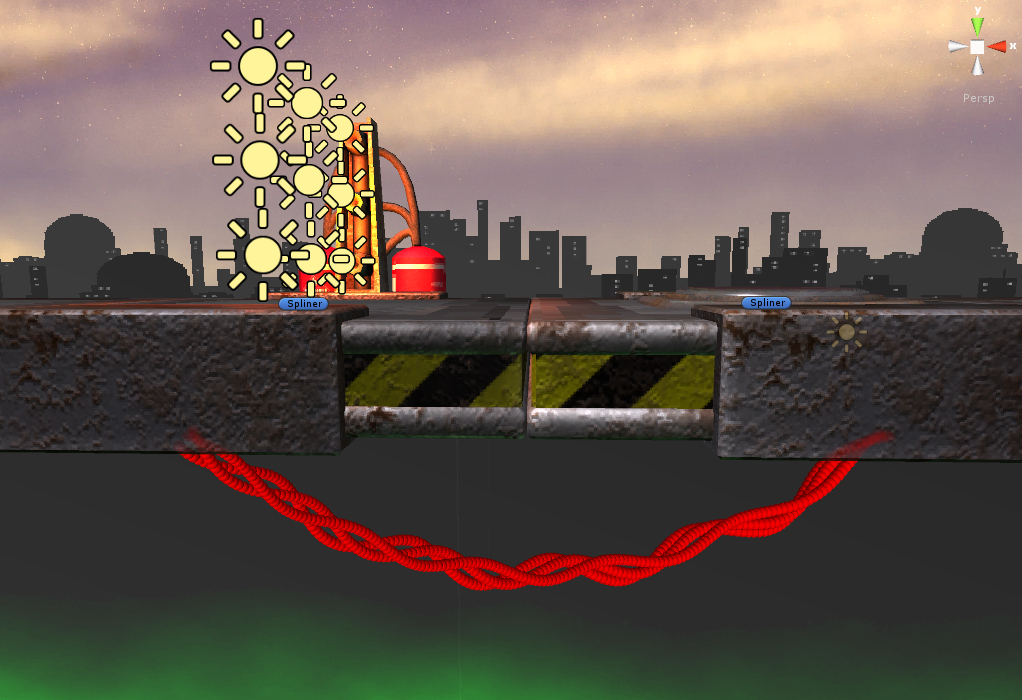## Procedural Splines

For my current project I need some way to show the player which button does what. The easiest and way that doesn’t show too much or annoy the player is some wires connecting the buttons to their respective mechanisms. First I created some models, but I wanted more, so I decided to write some code to procedurally create wires between two objects.

I divided the problem into three smaller problems:

• create a Master Spline between two objects that curves according to gravity (cosh(x))
• create multiple sub-Splines that curl around the Master Spline
• create the actual mesh by creating and connecting vertices around the sub-Splines

Master Spline:

For this I first had to write a cosh(x) function, because Unity doesn’t have one. That was easy thanks to Wikipedia and the almighty exponential function. Then I took the distance between the two objects and divided it into discrete steps and calculated the cosh for each of these steps. This I used to calculate positions that follow the cosh function between the two objects, no matter how I place them in XYZ space. The influence of gravity and number of steps can be easily controlled via public variables.

```using UnityEngine;
using System.Collections;

[ExecuteInEditMode]
public class Spliner : MonoBehaviour {

public GameObject next;
public float gravity;
public int steps;

private Vector3 nextPosition;
private Vector3[] pointArr;

void Update() {

if(steps < 0) steps= 0;
pointArr = new Vector3[steps];

if(next) nextPosition = next.transform.position;

for(int i = 0; i < steps; i++) {
pointArr[i] = Midpoint(i);
}
}

Vector3 Midpoint(int i) {
Vector3 direction = nextPosition - transform.position;

return transform.position + direction/steps * i + new Vector3(0, gravity * ((CosH((1.0f * i / steps) - 0.5f) - CosH(0.5f))), 0);
}

float CosH(float t) {
return (Mathf.Exp(t) + Mathf.Exp(-t))/2;
}

void OnDrawGizmos() {
foreach(Vector3 temp in pointArr) {
Gizmos.DrawSphere(temp, 0.1f);
}
}
}
```

Sub-Spline:
This was a bit more difficult. I used the points calculated for the Master Spline and calculated a cosine-sine spiral around them to make the sub-Splines curl around the Master Spline. I also added some randomness to frequency, amplitude and start-point of the sin/cos functions for each sub-Spline.

```using UnityEngine;
using System.Collections;

[ExecuteInEditMode]
public class Spliner : MonoBehaviour {

public GameObject next;
public float gravity;
public int sides;
public int steps;

public int subSplines;
public float frequency;
public float amplitude;

private Vector3 curPosition;
private Vector3 nextPosition;
private Vector3[] mainPointArr = new Vector3;

private Vector3[,] subPointArr = new Vector3[0,0];

void Update() {
if(next) {
nextPosition = next.transform.position - 2.8f * next.transform.forward;    //the offset here and in the next line are optional, they are important for my current project only
curPosition = transform.position - 2.8f * transform.forward;

if(sides < 3) sides = 3;

if(steps < 1) steps= 1;

mainPointArr = new Vector3[steps + 1];

for(int i = 0; i <= steps; i++) {
mainPointArr[i] = DrawCosH(i);
}

if(subSplines < 1) subSplines = 1;

subPointArr = new Vector3[subSplines, steps];

for(int i = 0; i < subSplines; i++) {
DrawSubSpline(i);
}
}
}

//create master spline
Vector3 DrawCosH(int i) {
Vector3 direction = nextPosition - curPosition;

return curPosition + direction/steps * i + new Vector3(0, gravity * (CosH((1.0f * i / steps) - 0.5f) - CosH(0.5f)), 0) - Vector3.up;    //this last Vector3.up is optional, like the offset at the top
}

//calculate cosh
float CosH(float t) {
return (Mathf.Exp(t) + Mathf.Exp(-t))/2;
}

//create sub splines
void DrawSubSpline(int i) {
float locAmp = amplitude * Random.Range(0.5f, 1.2f) / 10;
float locFreq = frequency * Random.Range(0.8f, 1.2f);
float offset = Random.Range(0.0f, 1.0f);
int cw = (Random.Range(0.0f, 1.0f) > 0.5 ? -1 : 1);

for(int j = 0; j < steps; j++) {
Vector3 direction = mainPointArr[j + 1] - mainPointArr[j];
Quaternion rot = Quaternion.LookRotation(direction);

subPointArr[i, j] = mainPointArr[j] + rot * (Vector3.up * Mathf.Sin(1.0f * j * locFreq / steps + offset) + Vector3.right * cw * Mathf.Cos(1.0f * j * locFreq / steps + offset)) * locAmp;
}
}

//draw Gizmos for visualization in the Editor
void OnDrawGizmos() {
if(next) {
Gizmos.color = Color.yellow;
//foreach(Vector3 temp in mainPointArr) {
//   Gizmos.DrawSphere(temp, 0.1f);
//}

if(subSplines > 0) {
Gizmos.color = Color.red;
foreach(Vector3 temp in subPointArr) {
Gizmos.DrawSphere(temp, 0.05f);
}
}
}
}
}
```

Now I have to read into creating meshes in code. Hopefully I will be able to finish this soon.

But first an image showing how the wires look like currently (for this I calculated many steps and drew a sphere gizmo for each of the points).